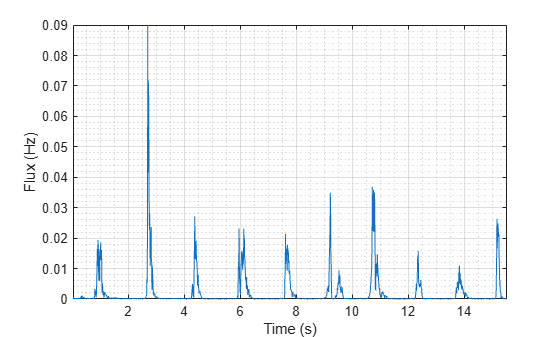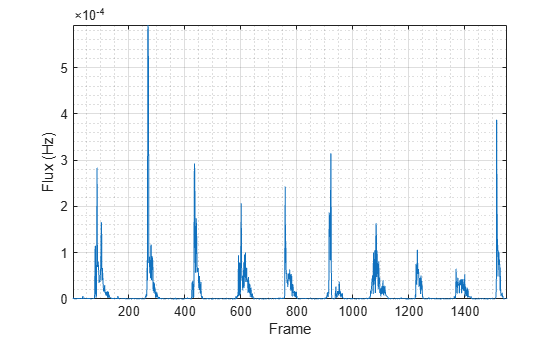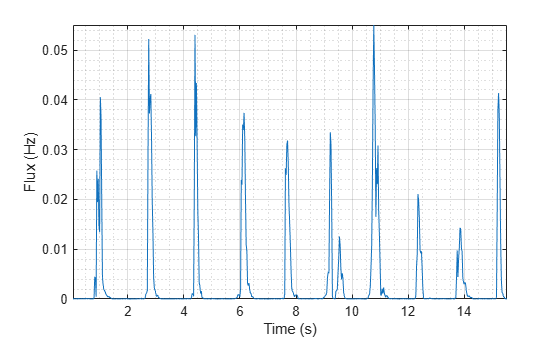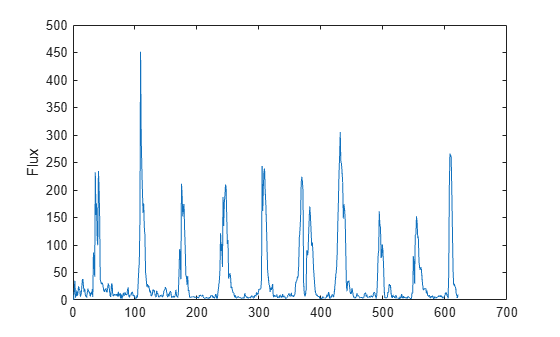# spectralFlux

Spectral flux for audio signals and auditory spectrograms

## Syntax

``flux = spectralFlux(x,f)``
``flux = spectralFlux(x,f,initialCondition)``
``flux = spectralFlux(___,Name=Value)``
``[flux,finalCondition] = spectralFlux(___)``
``spectralFlux(___)``

## Description

example

````flux = spectralFlux(x,f)` returns the spectral flux of the signal, `x`, over time. Spectral flux is a measure of the variability of the spectrum over time. How the function interprets `x` depends on the shape of `f`.```

example

````flux = spectralFlux(x,f,initialCondition)` specifies the previous spectral state. This syntax is supported only for frequency-domain inputs.```

example

````flux = spectralFlux(___,Name=Value)` specifies options using one or more name-value arguments.For example, `flux = spectralFlux(x,f,NormType=1)` calculates spectral flux using norm type 1.```

example

````[flux,finalCondition] = spectralFlux(___)` also returns the final spectral state. You can specify an input combination from any of the previous syntaxes.```

example

````spectralFlux(___)` with no output arguments plots the spectral flux. If the input is in the time domain, the spectral flux is plotted against time.If the input is in the frequency domain, the spectral flux is plotted against frame number. ```

## Examples

collapse all

Read in an audio file and calculate the flux using default parameters.

```[audioIn,fs] = audioread("Counting-16-44p1-mono-15secs.wav"); flux = spectralFlux(audioIn,fs);```

Plot the spectral flux against time.

`spectralFlux(audioIn,fs)`Read in an audio file and then calculate the mel spectrogram using the `melSpectrogram` function. Calculate the flux of the mel spectrogram over time.

```[audioIn,fs] = audioread("Counting-16-44p1-mono-15secs.wav"); [s,cf,t] = melSpectrogram(audioIn,fs); flux = spectralFlux(s,cf);```

Plot the spectral flux against the frame number.

`spectralFlux(s,cf)``[audioIn,fs] = audioread("Counting-16-44p1-mono-15secs.wav");`

Calculate the flux of the power spectrum over time. Calculate the flux for 50 ms Hamming windows of data with 25 ms overlap. Use the range from 62.5 Hz to `fs`/2 for the flux calculation.

```flux = spectralFlux(audioIn,fs, ... Window=hamming(round(0.05*fs)), ... OverlapLength=round(0.025*fs), ... Range=[62.5,fs/2]); ```

Plot the spectral flux.

```spectralFlux(audioIn,fs, ... Window=hamming(round(0.05*fs)), ... OverlapLength=round(0.025*fs), ... Range=[62.5,fs/2])```Spectral flux measures the change in consecutive spectra. To calculate spectral flux of streaming audio, you can pass the state in and out of the function.

Create a `dsp.AudioFileReader` object to read in audio data frame-by-frame. Create a `dsp.AsyncBuffer` object to buffer the audio input into overlapped frames. Create a second `dsp.AsyncBuffer` object to log the spectral flux calculation.

```fileReader = dsp.AudioFileReader('Counting-16-44p1-mono-15secs.wav'); inputBuffer = dsp.AsyncBuffer; logger = dsp.AsyncBuffer;```

In an audio stream loop:

2. Write the audio data to the input buffer.

3. If a hop of data is available from the buffer, read a frame of data with overlap.

4. Calculate the one-sided magnitude short time Fourier transform.

5. Calculate the spectral flux.

6. Log the spectral flux for later plotting.

```fs = fileReader.SampleRate; samplesPerFrame = round(fs*0.05); samplesOverlap = round(fs*0.025); samplesPerHop = samplesPerFrame - samplesOverlap; win = hamming(samplesPerFrame,'periodic'); Sprev = []; while ~isDone(fileReader) audioIn = fileReader(); write(inputBuffer,audioIn); while inputBuffer.NumUnreadSamples >= samplesPerHop audioBuffered = read(inputBuffer,samplesPerFrame,samplesOverlap); [S,f] = stft(audioBuffered,fs,"Window",win,"OverlapLength",samplesOverlap,"FrequencyRange","onesided"); [flux,Sprev] = spectralFlux(abs(S),f,Sprev); write(logger,flux); end end release(fileReader)```

Plot the logged data.

```plot(read(logger)) ylabel('Flux')```## Input Arguments

collapse all

Input signal, specified as a vector, matrix, or 3-D array. How the function interprets `x` depends on the shape of `f`.

Data Types: `single` | `double`

Sample rate or frequency vector in Hz, specified as a scalar or vector, respectively. How the function interprets `x` depends on the shape of `f`:

• If `f` is a scalar, `x` is interpreted as a time-domain signal, and `f` is interpreted as the sample rate. In this case, `x` must be a real vector or matrix. If `x` is specified as a matrix, the columns are interpreted as individual channels.

• If `f` is a vector, `x` is interpreted as a frequency-domain signal, and `f` is interpreted as the frequencies, in Hz, corresponding to the rows of `x`. In this case, `x` must be a real L-by-M-by-N array, where L is the number of spectral values at given frequencies of `f`, M is the number of individual spectra, and N is the number of channels.

Data Types: `single` | `double`

Previous spectral state, specified as an L-by-N matrix, where:

• L is the number of bins in the one-sided spectral representation, equal to `numel(f)`.

• N is the number of channels of audio data, equal to `size(x,3)`.

If `initialCondition` is unspecified, or specified as an empty, `spectralFlux` considers the first spectrum as repeating. That is, the first `flux` output is zero.

#### Dependencies

This input argument is only valid if the input, `x`, is a frequency-domain representation of audio. The `spectralFlux` function interprets the domain of the input `x` based on the size of `f`.

Data Types: `single` | `double`

### Name-Value Arguments

Specify optional pairs of arguments as `Name1=Value1,...,NameN=ValueN`, where `Name` is the argument name and `Value` is the corresponding value. Name-value arguments must appear after other arguments, but the order of the pairs does not matter.

Before R2021a, use commas to separate each name and value, and enclose `Name` in quotes.

Example: `Window=hamming(256)`

Norm type used to calculate flux, specified as `2` or `1`.

Data Types: `single` | `double`

Note

The following name-value arguments apply if `x` is a time-domain signal. If `x` is a frequency-domain signal, the following name-value arguments are ignored.

Window applied in the time domain, specified as a real vector. The number of elements in the vector must be in the range [1, `size(x,1)`]. The number of elements in the vector must also be greater than `OverlapLength`.

Data Types: `single` | `double`

Number of samples overlapped between adjacent windows, specified as an integer in the range [0, `size(Window,1)`).

Data Types: `single` | `double`

Number of bins used to calculate the DFT of windowed input samples, specified as a positive scalar integer. If unspecified, `FFTLength` defaults to the number of elements in the `Window`.

Data Types: `single` | `double`

Frequency range in Hz, specified as a two-element row vector of increasing real values in the range [0, `f`/2].

Data Types: `single` | `double`

Spectrum type, specified as `"power"` or `"magnitude"`:

• `"power"` –– The spectral flux is calculated for the one-sided power spectrum.

• `"magnitude"` –– The spectral flux is calculated for the one-sided magnitude spectrum.

Data Types: `char` | `string`

## Output Arguments

collapse all

Spectral flux in Hz, returned as a scalar, vector, or matrix. Each row of `flux` corresponds to the spectral flux of a window of `x`. Each column of `flux` corresponds to an independent channel.

Final spectral state, returned as an L-by-N matrix, where:

• L is the number of bins in the one-sided spectral representation, equal to `numel(f)`.

• N is the number of channels of audio data, equal to `size(x,3)`.

#### Dependencies

This output argument is only valid if the input, `x`, is a frequency-domain representation of audio. The `spectralFlux` function interprets the domain of the input `x` based on the size of `f`.

## Algorithms

The spectral flux is calculated as described in :

`$\text{flux(}t\text{)}={\left(\sum _{k={b}_{1}}^{{b}_{2}}{|{s}_{k}\left(t\right)-{s}_{k}\left(t-1\right)|}^{P}\right)}^{1}{P}}$`

where

• sk is the spectral value at bin k.

• b1 and b2 are the band edges, in bins, over which to calculate the spectral flux.

• P is the norm type. You can specify the norm type using `NormType`.

 Scheirer, E., and M. Slaney. "Construction and Evaluation of a Robust Multifeature Speech/Music Discriminator." IEEE International Conference on Acoustics, Speech, and Signal Processing. Volume 2, 1997, pp. 1221–1224.